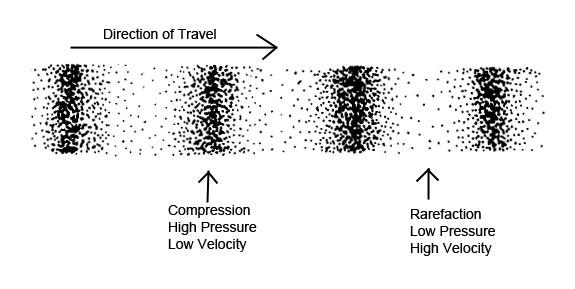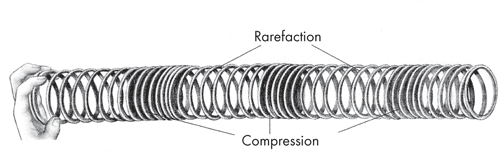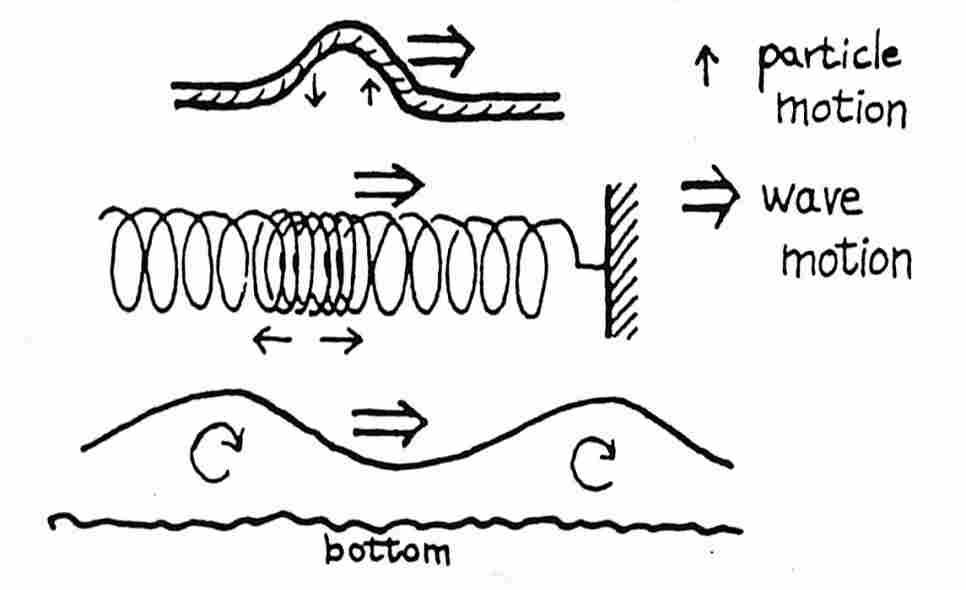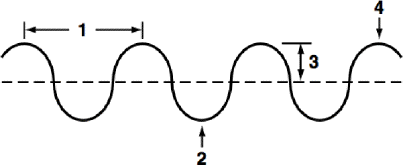# Mechanical Waves, Sound & the Electromagnetic Spectrum

 Mechanical Waves, Sound & the Electromagnetic Spectrum Nam e:____________________________ Date: ____ Chapter 11 & 12 Period: _____ Part I: Mechanical Waves (pg318-325) How are mechanical waves produced? What is a medium? Which of the following are mediums through which waves can travel? Circle all that apply. Rock Water Air A transverse wave causes the medium to vibrate at ____________________ angles, or perpendicular, to the direction of the wave. Draw perpendicular lines. What is an example of a transverse wave? A longitudinal wave is a wave in which the vibration of the medium is ____________________________________ to the direction the wave travels. Draw parallel lines. What is an example of a transverse wave? What is a compression? What is a rarefaction? Label the compressions & rarefactions of each wave. Are these longitudinal or transverse waves?What is a surface wave? Give an example. Label the mechanical waves in the diagram below.Label the parts of the wave (Fig 2): d. a._______________________________Fig 2 a. b. ______________________________ c. c. ______________________________ d. ______________________________ b.. What is the definition for wavelength? What is the definition for frequency? What is the definition for amplitude? What is the relationship between wavelength and frequency? How are the amplitude and pitch related?